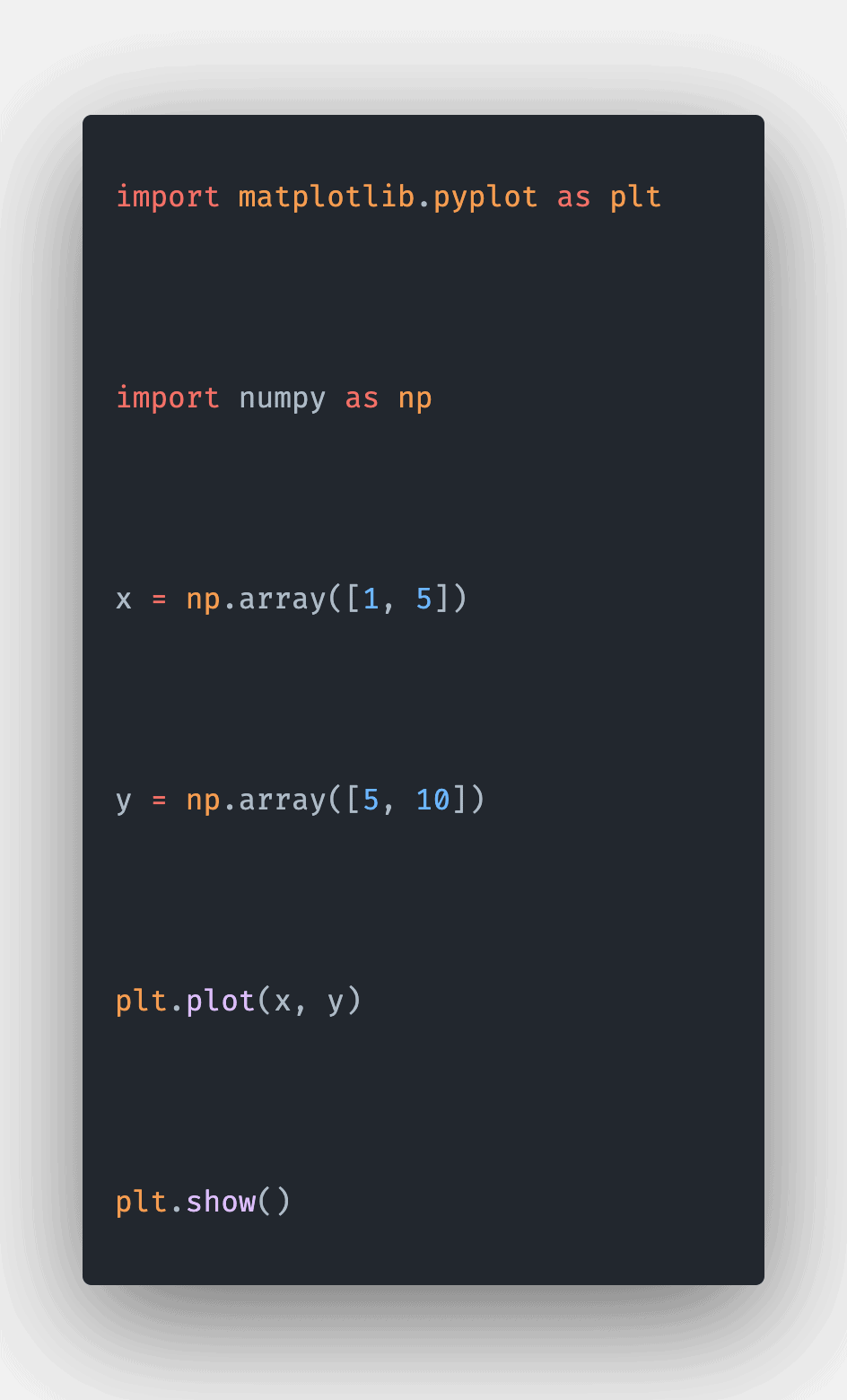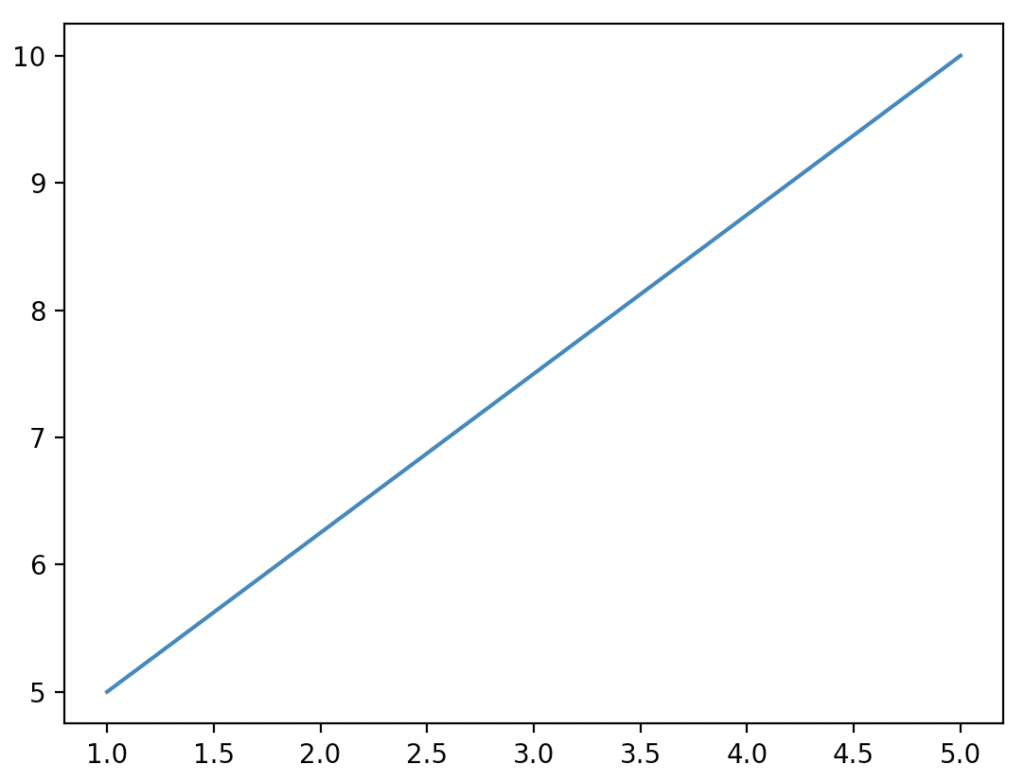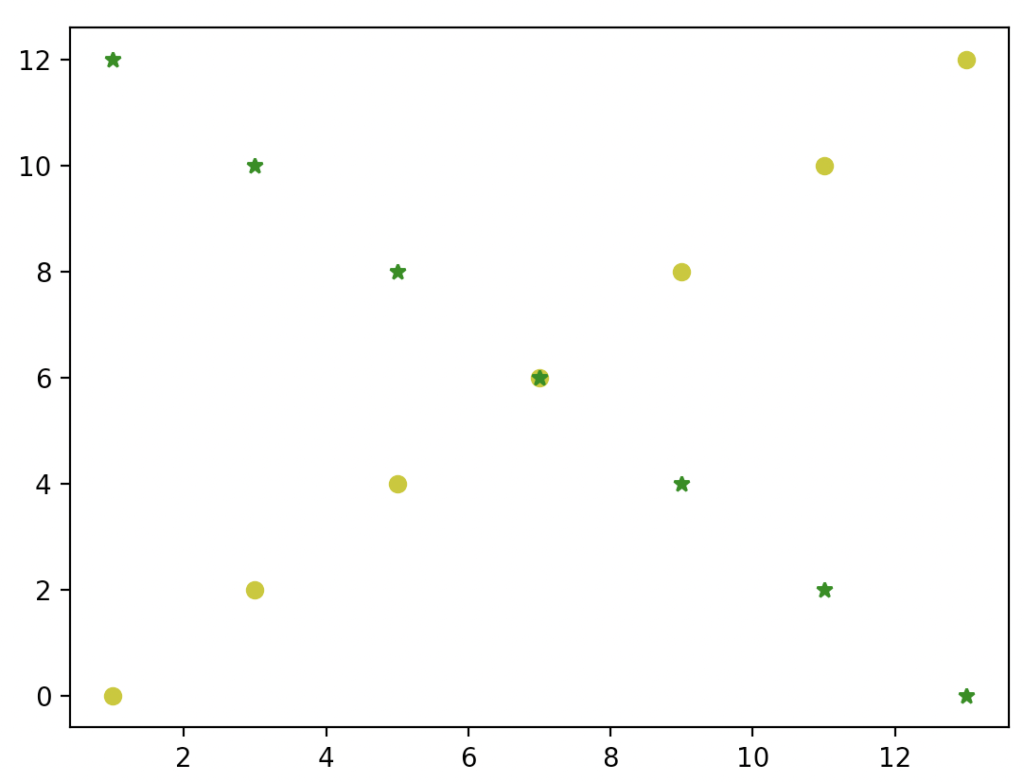# Matplotlib plot: The Complete Guide

0
3Matplotlib is a plotting library to display graphs and it is a numerical – mathematical extension for the Numpy library. Pyplot is a state-based interface to a Matplotlib module that provides a MATLAB-like interface. Let’s see the matplotlib.pyplot.plot() function in depth.

## matplotlib plot

The matplotlib.pyplot.plot() is a built-in library function that creates a graph for the given points and displays the graph as the output. The matplotlib.plot() function plots y versus x as lines and markers.

### Syntax

``matplotlib.pyplot.plot(*args, scalex=True, scaley=True, data=None, **kwargs)``

### Parameters

The matplotlib.pyplot.plot() function has one required argument and four optional arguments  as parameters:

1. args – x,y: This parameter has two values, x, and y. The points are passed in the x and y argument. This x and y can have values like numbers or a list of numbers.
2. fmt: fmt stands for format. This is an optional argument. This argument takes a string as the argument. This string represents the format in which the graph must be created. For example, some of the color codes used in string formats are r for red, b for blue, g for green, and y for yellow. Likewise, some of the markers used in the string formats are “o” for circle, “.” for point, “*” for star, and “+” for plus.
3. data: An object with labeled data is passed as an argument. This is an optional argument.

### Return value

The matplotlib.pyplot.lib() function returns a list of 2d lines representing the plotted data.

## Program for creating a plotted graph using matplotlib.pyplot.plot

``````# Importing matplotlib.pyplot as plt.
import matplotlib.pyplot as plt

# Importing numpy as np
import numpy as np

# create a numpy array for storing the x coordinates
x = np.array([1, 5])

# create a numpy array for storing the y coordinates
y = np.array([5, 10])

# pass the x coordinates and y coordinates into the plot() function
plt.plot(x, y)

# displaying the created graph using the show method
plt.show()``````

#### OutputWe imported matplotlib.pyplot() function for plotting the graph in this program. The matplotlib library consists of all the functions for plotting different types of graphs. Then we imported the numpy library for creating x and y coordinates.

In the next step, we created x and y coordinates and stored this numpy array in x and y variables. x variable stores the values from 1 to 5, and the y variable stores the values from 5 to 10. Then we have passed the two coordinates into the plot() function.

This function plots the graph across the x and y-axis. Then, the graph is displayed using the show() function.

## Program for creating two plotted graphs using matplotlib.pyplot.plot

``````# Importing matplotlib.pyplot as plt.
import matplotlib.pyplot as plt

# Importing numpy as np
import numpy as np

# create a numpy array for storing the x coordinates
x = np.array([1, 3, 5, 7, 9, 11, 13])

# create a numpy array for storing the y coordinates
y = np.array([0, 2, 4, 6, 8, 10, 12])

# x and y coorindates for the second line is stored in the variable
u = np.array([13, 11, 9, 7, 5, 3, 1])
v = np.array([0, 2, 4, 6, 8, 10, 12])

# the plot function is called with coordinates x,y,u,v
plt.plot(x, y, 'yo', u, v, 'g*')

# displaying the created graph using the show method
plt.show()``````

#### OutputIn this program, we imported matplotlib.pyplot for plotting the graph. The matplotlib library consists of all the functions for plotting different types of graphs. Then we imported numpy for creating x and y coordinates.

In the next step, we created x and y coordinates and stored this numpy array in x and y variables.

Then, we created the x and y coordinates for the second line and stored them in the u and v variables.

Then we called the plot function with x and y as the first argument then we have given the format of the line as a yellow circle.

At last, we passed the second line coordinates and the format of the string is passed as a green star.

That’s it for this tutorial.

This site uses Akismet to reduce spam. Learn how your comment data is processed.